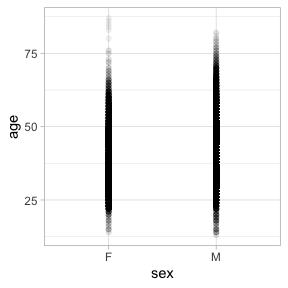# Formula-driven graphics

There are several excellent graphics packages provided for R. The `ggformula` package currently builds on one of them, `ggplot2`, but provides a very different user interface for creating plots. The interface is based on formulas (much like the `lattice` interface) and the use of the chaining operator (`%>%`) to build more complex graphics from simpler components.

The `ggformula` graphics were designed with several user groups in mind:

• beginners who want to get started quickly and may find the syntax of `ggplot2()` a bit offputting,

• those familiar with `lattice` graphics, but wanting to be able to easily create multilayered plots,

• those who prefer a formula interface, perhaps because it is familiar from use with functions like `lm()` or from use of the `mosaic` package for numerical summaries.

## The basic formula template

The basic template for creating a plot with `ggformula` is

``gf_plottype(formula, data = mydata)``

where

• `plottype` describes the type of plot (layer) desired (points, lines, a histogram, etc., etc.),

• `mydata` is a data frame containing the variables used in the plot, and

• `formula` describes how/where those variables are used.

For example, in a bivariate plot, `formula` will take the form `y ~ x`, where `y` is the name of a variable to be plotted on the y-axis and `x` is the name of a variable to be plotted on the x-axis. (It is also possible to use expressions that can be evaluated using variables in the data frame as well.)

Here is a simple example:

``````library(ggformula)
gf_point(mpg ~ hp, data = mtcars)``````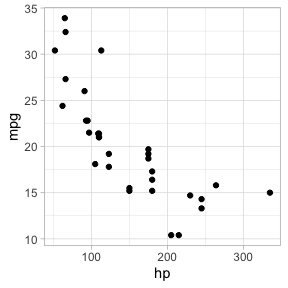## Selecting the glyph type

The “kind of graphic” is specified by the name of the graphics function. All of the `ggformula` data graphics functions have names starting with `gf_`, which is intended to remind the user that they are formula-based interfaces to `ggplot2`: `g` for `ggplot2` and `f` for “formula.” Commonly used functions include

• `gf_point()` for scatter plots
• `gf_line()` for line plots (connecting dots in a scatter plot)
• `gf_density()` or `gf_dens()` or `gf_histogram()` or `gf_dhistogram()` or `gf_freqpoly()` to display distributions of a quantitative variable
• `gf_boxplot()` or `gf_violin()` for comparing distributions side-by-side
• `gf_counts()` for bar-graph style depictions of counts.
• `gf_bar()` for more general bar-graph style graphics

The function names generally match a corresponding function name from `ggplot2`, although

• `gf_counts()` is a simplified special case of `geom_bar()`,
• `gf_dens()` is an alternative to `gf_density()` that displays the density plot slightly differently
• `gf_dhistogram()` produces a density histogram rather than a count histogram.

Each of the `gf_` functions can create the coordinate axes and fill it in one operation. (In `ggplot2` nomenclature, `gf_` functions create a frame and add a layer, all in one operation.) This is what happens for the first `gf_` function in a chain. For subsequent `gf_` functions, new layers are added, each one “on top of” the previous layers.

## Attributes

Each of the marks in the plot is a glyph. Every glyph has graphical attributes (called aesthetics in `ggplot2`) that tell where and how to draw the glyph. In the above plot, the obvious attributes are x- and y-position:
We’ve told R to put `mpg` along the y-axis and `hp` along the x-asis, as is clear from the plot.

But each point also has other attributes, including color, shape, size, stroke, fill, and alpha (transparency). We didn’t specify those in our example, so `gf_point()` uses some default values for those – in this case smallish black filled-in circles.

### Specifying attributes

In the `gf_` functions, you specify the non-position graphical attributes using an extension of the basic formula. Attributes can be set to a constant value (e.g, set the color to “blue”; set the size to 2) or they can be mapped to a variable in the data or some expression involving the variables (e.g., map the color to `sex`, so sex determines the color groupings)

Attributes are set or mapped using additional arguments.

• adding an argument of the form `attribute = value` sets `attribute` to `value`.
• adding an argument of the form `attribute = ~ expression` maps `attribute` to `expression`

where `attribute` is one of `color`, `shape`, etc., `value` is a constant (e.g. `"red"` or `0.5`, as appropriate), and `expression` may be some more general expression that can be computed using the variables in `data` (although often is is better to create a new variable in the data and to use that variable instead of an on-the-fly calculation within the plot).

The following plot, for instance,

• We use `cyl` to determine the color and `carb` to determine the size of each dot. Color and size are mapped to `cyl` and `carb`. A legend is provided to show us how the mapping is being done. (Later, we can use scales to control precisely how the mapping is done – which colors and sizes are used to represent which values of `cyl` and `carb`.)

• We also set the transparency to 50%. The gives the same value of `alpha` to all glyphs in this layer.

``gf_point(mpg ~ hp, color = ~ cyl, size = ~ carb, alpha = 0.50, data = mtcars) ``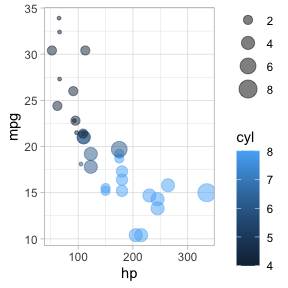### On-the-fly calculations

`ggformula` allows for on-the-fly calculations of attributes, although the default labeling of the plot is often better if we create a new variable in our data frame. In the examples below, since there are only three values for `carb`, it is easier to read the graph if we tell R to treat `cyl` as a categorical variable by converting to a factor (or to a string). Except for the labeling of the legend, these two plots are the same.

``````library(dplyr)
gf_point(mpg ~ hp,  color = ~ factor(cyl), size = ~ carb, alpha = 0.75, data = mtcars)
gf_point(mpg ~ hp,  color = ~ cylinders, size = ~ carb, alpha = 0.75,
data = mtcars %>% mutate(cylinders = factor(cyl)))``````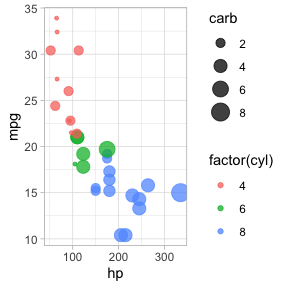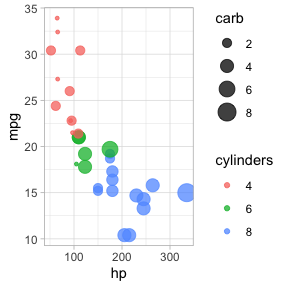## “One-variable” plots

For some plots, we only have to specify the x-position because the y-position is calculated from the x-values. Histograms, densityplots, and frequency polygons are examples. To illustrate, we’ll use density plots, but the same ideas apply to `gf_histogram()`, and `gf_freqpolygon()` as well. Note that in the one-variable density graphics, the variable whose density is to be calculated goes to the right of the tilde, in the position reserved for the x-axis variable.

``````data(Runners, package = "mosaicModel")
Runners <- Runners %>% filter( ! is.na(net))
gf_density( ~ net, data = Runners)
gf_density( ~ net,  fill = ~ sex,  alpha = 0.5, data = Runners)
# gf_dens() is similar, but there is no line at bottom/sides, and it is not "fillable"
gf_dens( ~ net, color = ~ sex, alpha = 0.7, data = Runners)    ``````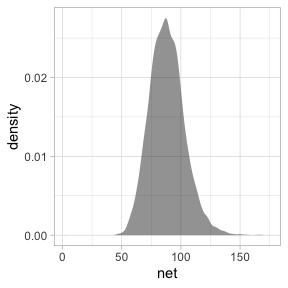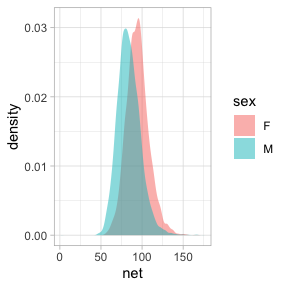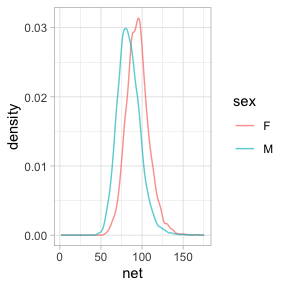Several of the plotting functions include additional arguments that do not modify attributes of individual glyphs but control some other aspect of the plot. In this case, `adjust` can be used to increase or decrease the amount of smoothing.

``````# less smoothing
gf_dens( ~ net, color = ~ sex, alpha = 0.7, data = Runners, adjust = 0.25)
# more smoothing
gf_dens( ~ net, color = ~ sex, alpha = 0.7, data = Runners, adjust = 4)     ``````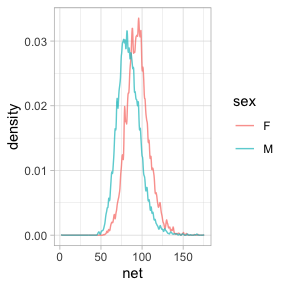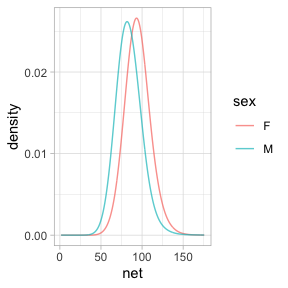## Position

When the `fill` or `color` or `group` aesthetics are mapped to a variable, the default behavior is to lay the group-wise densities on top of one another. Other behavior is also available by using `position` in the formula. Using the value `"stack"` causes the densities to be laid one on top of another, so that the overall height of the stack is the density across all groups. The value `"fill"` produces a conditional probability graphic.

``````gf_density( ~ net, fill = ~ sex, color = NA, position = "stack", data = Runners)
gf_density( ~ net, fill = ~ sex, color = NA, position = "fill", data = Runners, adjust = 2)``````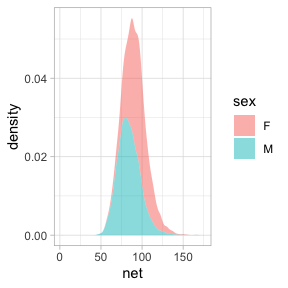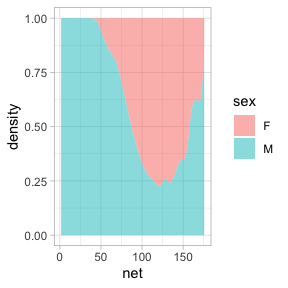Similar commands can be constructed with `gf_histogram()` and `gf_freqpoly()`, but note that `color`, not `fill`, is the active attribute for frequency polygons. It’s also rarely good to overlay histograms on top of one another – better to use a density plot or a frequency polygon for that application.

## Faceting

The `ggplot2` system allows you to make subplots — called “facets” — based on the values of one or two categorical variables. This is done by chaining with `gf_facet_grid()` or `gf_facet_wrap()`. These functions use formulas to specify which variable(s) are to be used for faceting.

``````gf_density_2d(net ~ age, data = Runners) %>% gf_facet_grid( ~ sex)
# the dot here is a bit strange, but required to make a valid formula
gf_density_2d(net ~ age, data = Runners) %>% gf_facet_grid( sex ~ .)
gf_density_2d(net ~ age, data = Runners) %>% gf_facet_wrap( ~ year)
gf_density_2d(net ~ age, data = Runners) %>% gf_facet_grid(start_position ~ sex)``````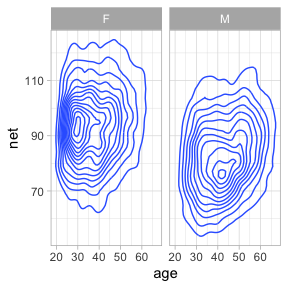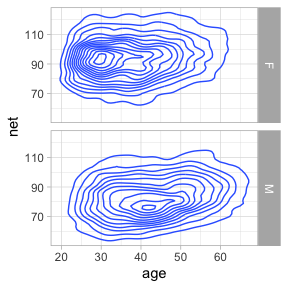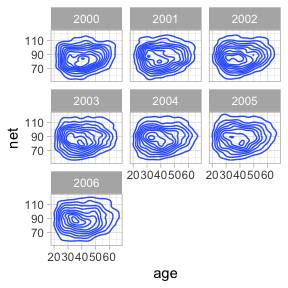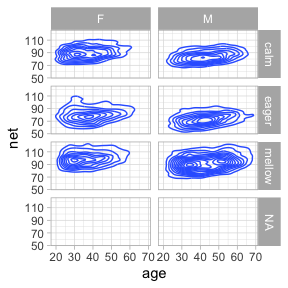An alternative syntax uses `|` to separate the faceting information from the main part of the formula.
Here is another example using our weather data. The redundant use of the `y` and `color` attributes for temperature makes it easier to compare across facets.

``gf_ribbon(low_temp + high_temp ~ date | city ~ year, data = Weather, alpha = 0.3) ``
``## Warning: Detecting old grouped_df format, replacing `vars` attribute by `groups```
``````gf_linerange(low_temp + high_temp ~ date | city ~ year, color = ~ avg_temp, data = Weather) %>%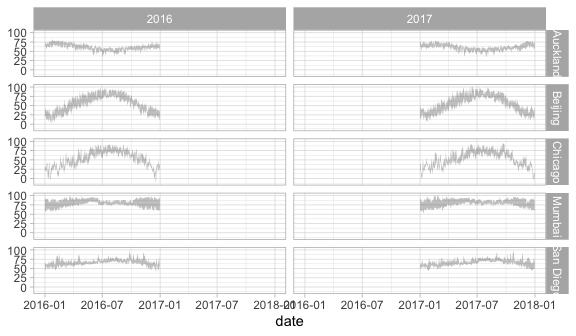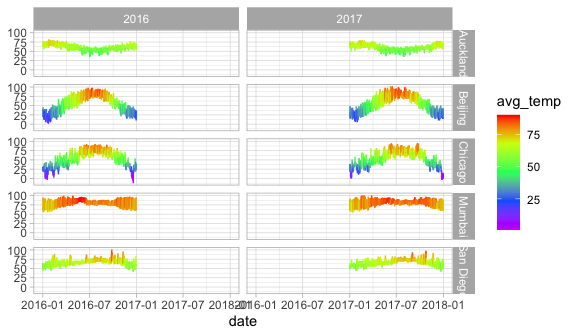In this case, we should either not facet by year, or allows the x-scale to be freely adjusted in each column so that we don’t have so much unnecessary white space. We can do the latter using the `scales` argument to `gf_facet_grid()`.

``````gf_ribbon(low_temp + high_temp ~ date | city ~ ., data = Weather, alpha = 0.3)

gf_linerange(low_temp + high_temp ~ date, color = ~ avg_temp, data = Weather) %>%
gf_facet_grid(city ~ year, scales = "free_x")``````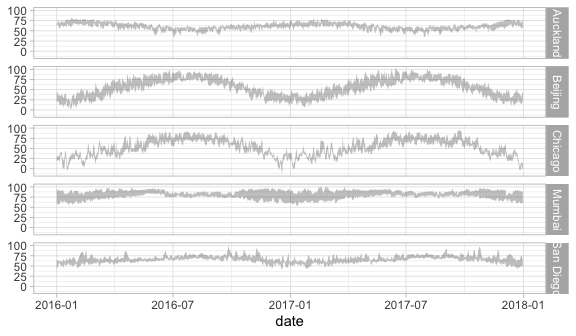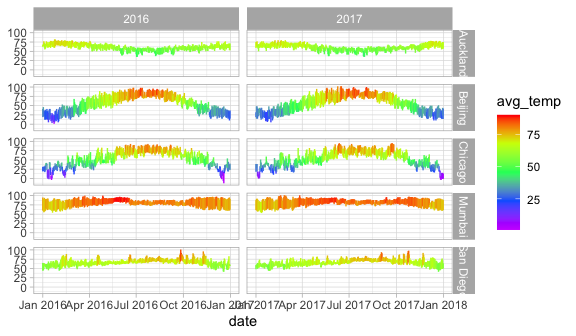## More 2-variable plots

### Using jitter and transparency to handle overlapping cases

Sometimes you have so many points in a scatter plot that they obscure one another. The `ggplot2` system provides two easy ways to deal with this: translucency and jittering.

Use `alpha = 0.5` to make the points semi-translucent. If there are many points overlapping at one point, a much smaller value of alpha, say `alpha = 0.01`. We’ve already seen this above.

Using `gf_jitter()` in place of `gf_point()` will move the plotted points to reduce overlap. Jitter and transparency can be used together as well.

``````gf_point(age ~ sex, alpha = 0.05, data = Runners)
gf_jitter(age ~ sex, alpha = 0.05, data = Runners)``````